# Draw Histogram with Logarithmic Scale in R

• Last Updated : 30 May, 2021

R is a programming language, mainly used for statistical computing and graphics. It implements various statistical techniques including linear modeling, classification, time series analysis, and clustering. A Histogram is commonly used to depict the frequency distribution of variables. It also is often used to summarize both continuous and discrete data. Unlike the bar chart where the frequency is indicated by the height of the bars, here the frequency is indicated by the area of the bar.

In this article, we will see how to draw a histogram with a Logarithmic scale in R Programming Language.

Method 1: Using Base R

The hist() function to create histograms.

Syntax:

Syntax : hist( v, main, xlab, xlim, ylim, breaks, col, border)

If we want to convert the values of our histogram to a logarithmic scale, we can use the log() function within the hist() function as shown below:

hist(log(v))

• v : The vector containing the numeric values to be used in histogram.

Example 1: Creating a Normal Histogram

A vector ‘A’ is taken which contains the numerical values to be used by the histogram. The vector ‘A‘ is plotted using the hist() function, the title of the plot is set to “Histogram” using the main parameter.

## R

 `# Code for Plotting A Normal Histogram``# Create data for the Histogram``A <- ``c``(10, 10, 10, 20, 15,``       ``15, 20, 20, 20, 20)`` ` `# Plot the histogram``hist``(A, main = ``"Histogram"``)`

Output: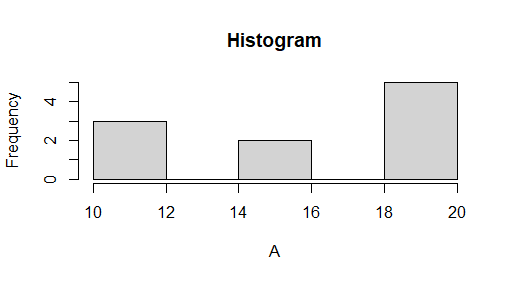Example 2: Creating a Histogram with Logarithmic Scale in R

A vector ‘A‘ is taken which contains the numerical values to be used by the histogram, the vector ‘A‘ is plotted using the log() function inside the hist() function.

The title of the plot is set to “Histogram”, the color of the bar is set to “green”, border color of the bar is set to “red” and the xlabel is set to “Logarithmic Scale” using the main, col, border, xlab parameters respectively.

Code:

## R

 `# Code for Plotting A Histogram in``# the Logarithmic Scale`` ` `# Create data for the Histogram``A <- ``c``(10, 10, 10, 20, 20,``       ``20, 20, 20, 30, 30)`` ` `# Plot the histogram``hist``(``log``(A), main = ``"Histogram"``,``     ``col = ``"green"``, border = ``"red"``,``     ``xlab = ``"Logarithmic Scale"``)`

Output: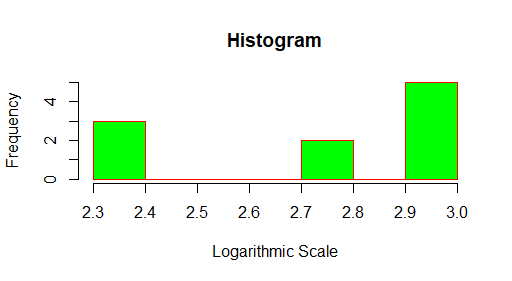Method 2: Using ggplot2 Package

Install ggplot2 package using the install.packages() and Load the ggplot2 package using the library().

```# Install & load ggplot2 package
install.packages("ggplot2")
library("ggplot2")```

The ggplot2 is an R package dedicated to data visualization. With the ggplot2 package, we can plot almost any type of chart.

Syntax: ggplot(data.frame(x), aes(x)) + geom_histogram()

• x is the vector which contains the numerical values to be used by the histogram.
• data.frame(x) creates a data frame from the given data
• aes(x) is often used within other graphing elements to specify the desired aesthetics
• geom_histogram() is used to plot the histogram

Creating a Normal Histogram:

1. A vector “A” is taken which contains the numerical values to be used by the histogram.
2. The histogram is plotted using the function ggplot() function and the geom_histogram() function

Example:

## R

 `library``(``"ggplot2"``)`` ` `# Code for Plotting A Histogram in ``# the Logarithmic Scale`` ` `# Create data for the Histogram``A <- ``c``(10, 10, 10, 20, 15,``       ``15, 20, 20, 20, 20)`` ` `# Plot the histogram``# The bins parameter divides the plot``# into 5 parts/bars``ggplot``(``data.frame``(A), ``aes``(A)) + ``geom_histogram``(bins = 5)`

Output: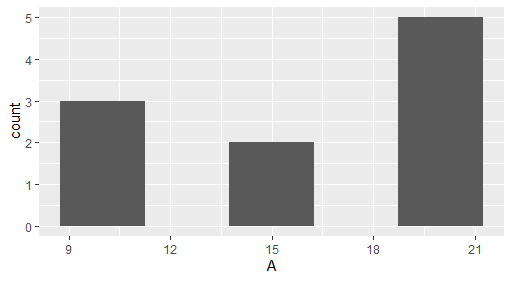Method 3: Using ggplot2 Package and log() function.

If we want to convert the values of our histogram to a logarithmic scale, we can use the log() function within the ggplot() and geom_histogram() function as shown below:

Syntax: ggplot(data.frame(log(x)), aes(log(x))) + geom_histogram()

• x is the vector which contains the numerical values to be used by the histogram.
• data.frame(log(x)) creates a data frame from log(x)
• aes(log(x)) is often used within other graphing elements to specify the desired aesthetics
• geom_histogram() is used to plot the histogram

A vector ‘A‘ is taken which contains the numerical values to be used by the histogram. Transform x-axis to logarithmic scale using log() function, plot the vector ‘A‘ using the ggplot() function, log() function and geom_histogram() function. The color of the bars is set to green and the border color is set to red using fill and color respectively.

## R

 `library``(``"ggplot2"``)`` ` ` ` `# Code for Plotting A Histogram in ``# the Logarithmic Scale`` ` `# Create data for the Histogram``A <- ``c``(10, 10, 10, 20, 15,``       ``15, 20, 20, 20, 20)`` ` `# Plot the histogram``# The bins parameter divides the plot ``# into 5 parts/bars``ggplot``(``data.frame``(``log``(A)),``       ``aes``(``log``(A))) + ``geom_histogram``(bins = 5)`

Output: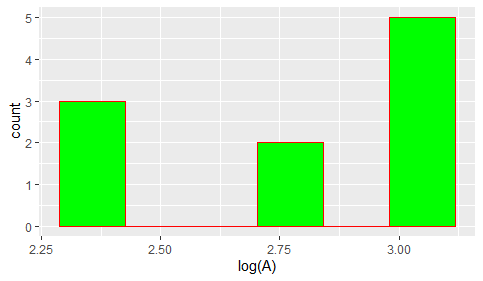Method 4: Using ggplot2 Package and scale_x_log10() function.

If we want to convert the values of our histogram to a logarithmic scale, we can use the scale_x_log10() function along with the ggplot() and geom_histogram() function as shown below:

Syntax: ggplot(data.frame(x), aes(x)) + geom_histogram() + scale_x_log10()

Parameters:

• x is the vector which contains the numerical values to be used by the histogram.
• data.frame(x) creates a data frame from x.
• aes(x) is often used within other graphing elements to specify the desired aesthetics
• geom_histogram() is used to plot the histogram
• scale_x_log10() is used to convert the x-axis to logarithmic scale

A vector ‘A‘ is taken which contains the numerical values to be used by the histogram. Plot the vector ‘A‘ using the ggplot() function, scale_x_log10() function and geom_histogram() function, The color of the bars is set to green and the border colour is set to red using fill and color respectively.

## R

 `library``(``"ggplot2"``)`` ` ` ` `# Code for Plotting A Histogram in ``# the Logarithmic Scale`` ` `# Create data for the Histogram``A <- ``c``(10, 10, 10, 20, 15,``       ``15, 20, 20, 20, 20)`` ` `# Plot the histogram``# The bins parameter divides the``# plot into 6 parts/bars``ggplot``(``data.frame``(A), ``aes``(A)) +``geom_histogram``(color = ``"red"``, fill = ``"green"``, bins = 6) +``scale_x_log10``()`

Output: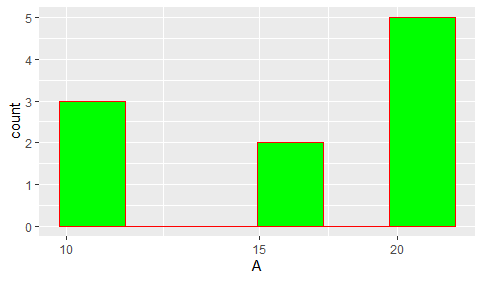My Personal Notes arrow_drop_up